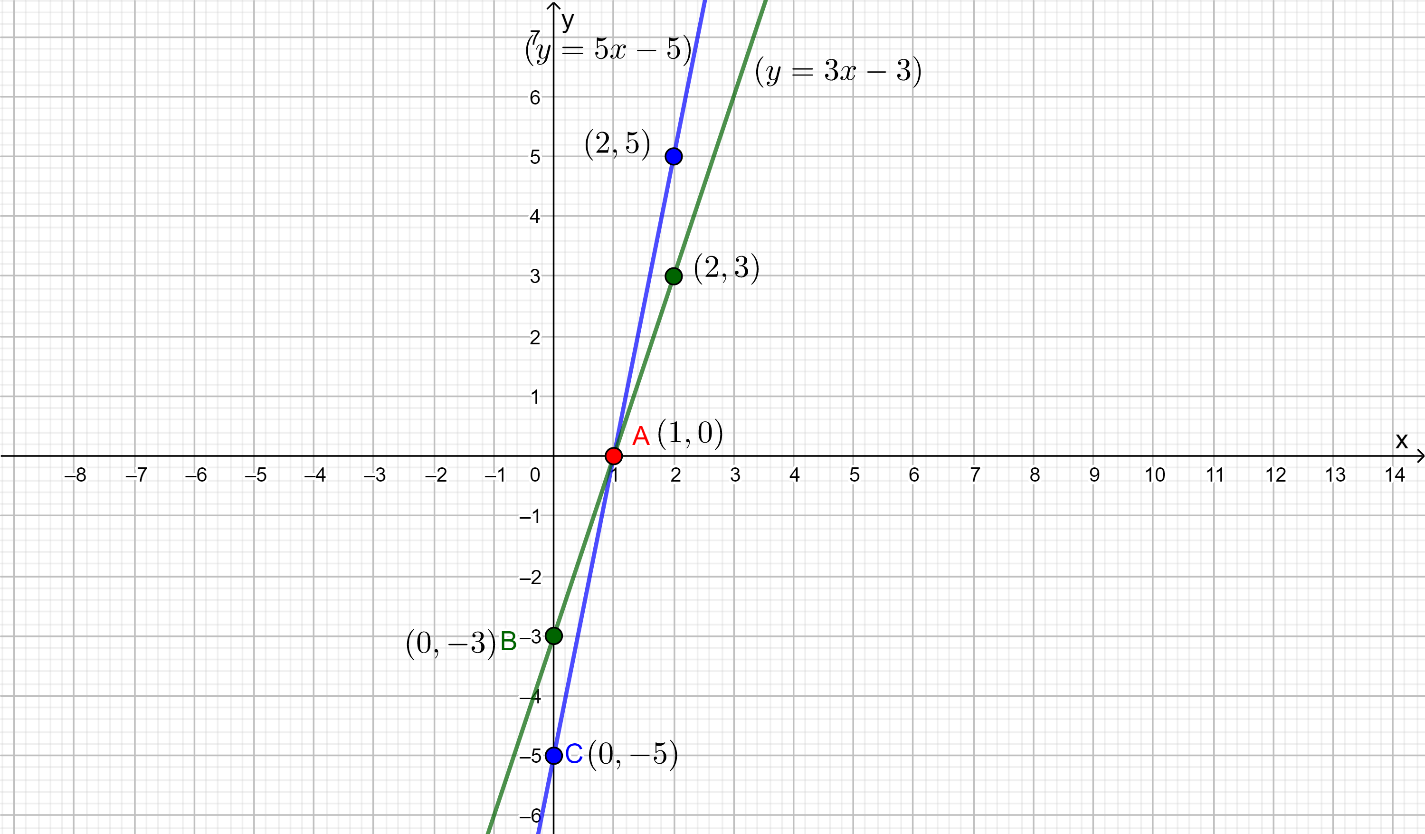# Ex.3.7 Q6 Pair of Linear Equations in Two Variables Solution - NCERT Maths Class 10

Go back to  'Ex.3.7'

## Question

Draw graphs of the equations $$5x - y = 5$$ and $$3x - y = 3$$. Determine the coordinates of the vertices of the triangle formed by these lines and the $$y$$ axis.

Video Solution
Pair Of Linear Equations In Two Variables
Ex 3.7 | Question 6

## Text Solution

Steps:

\begin{align}5x - y &= 5\\\Rightarrow \quad y &= 5x - 5\end{align}

The solution table will be as follows.

 $$x$$ $$0$$ $$2$$ $$y$$ $$-5$$ $$5$$

\begin{align}3x - y &= 3\\ \Rightarrow \quad y &= 3x - 3\end{align}

The solution table will be as follows.

 $$x$$ $$0$$ $$2$$ $$y$$ $$-3$$ $$3$$

The graphical representation of these lines will be as follows.It can be observed that the required triangle is $$ABC$$ formed by these lines and $$y-$$axis.

The coordinates of vertices are $$A( 1, 0),\; B (0, - 3),\; C (0, - 5)$$.

Learn from the best math teachers and top your exams

• Live one on one classroom and doubt clearing
• Practice worksheets in and after class for conceptual clarity
• Personalized curriculum to keep up with school